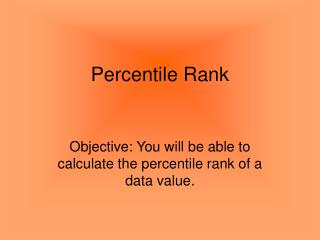DownloadDownload PresentationPercentile Rank

# Percentile Rank

Télécharger la présentation## Percentile Rank

- - - - - - - - - - - - - - - - - - - - - - - - - - - E N D - - - - - - - - - - - - - - - - - - - - - - - - - - -
##### Presentation Transcript

1. Percentile Rank Objective: You will be able to calculate the percentile rank of a data value.

2. A percentile is a measure that tells us what percent of the total frequency scored at or below that measure.

3. Formula: • To find the percentile rank of a score, x, out of a set of n scores, where x is included: Where B = number of scores below x            E = number of scores equal to x            n = number of scores

4. Key Points: • Percentile rank is a number between 0 and 100 indicating the percent of cases falling at or below that score. • Percentile ranks are usually written to the nearest whole percent:   74.5% = 75% = 75th percentile • Scores are arranged in rank order from lowest to highest • There is no 0 percentile rank - the lowest score is at the first percentile • There is no 100th percentile - the highest score is at the 99th percentile

5. Practice Problems

6. More challenging: